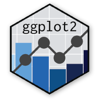Show Sidebar Hide Sidebar# geom_polygon in ggplot2

Examples of geom_polygon in R.

### New to Plotly?

Plotly's R library is free and open source!
You can set up Plotly to work in online or offline mode.
We also have a quick-reference cheatsheet (new!) to help you get started!

### Version Check

Version 4 of Plotly's R package is now available!
Check out this post for more information on breaking changes and new features available in this version.

``````library(plotly)
packageVersion('plotly')
``````
``````##  '4.9.1'
``````

### Basic Ploygon

``````library(plotly)

ids <- factor(c("1.1", "2.1", "1.2", "2.2", "1.3", "2.3"))

values <- data.frame(
id = ids,
value = c(3, 3.1, 3.1, 3.2, 3.15, 3.5)
)

positions <- data.frame(
id = rep(ids, each = 4),
x = c(2, 1, 1.1, 2.2, 1, 0, 0.3, 1.1, 2.2, 1.1, 1.2, 2.5, 1.1, 0.3,
0.5, 1.2, 2.5, 1.2, 1.3, 2.7, 1.2, 0.5, 0.6, 1.3),
y = c(-0.5, 0, 1, 0.5, 0, 0.5, 1.5, 1, 0.5, 1, 2.1, 1.7, 1, 1.5,
2.2, 2.1, 1.7, 2.1, 3.2, 2.8, 2.1, 2.2, 3.3, 3.2)
)

datapoly <- merge(values, positions, by=c("id"))

p <- ggplot(datapoly, aes(x=x, y=y)) + geom_polygon(aes(fill=value, group=id))

p <- ggplotly(p)

p
``````

Inspired by ggplot2 docs

### Ellipses

``````# create data
set.seed(20130226)
n <- 200
x1 <- rnorm(n, mean = 2)
y1 <- 1.5 + 0.4 * x1 + rnorm(n)
x2 <- rnorm(n, mean = -1)
y2 <- 3.5 - 1.2 * x2 + rnorm(n)
class <- rep(c("A", "B"), each = n)
df <- data.frame(x = c(x1, x2), y = c(y1, y2), colour = class)

# get code for "stat_ellipse"
library(devtools)
library(ggplot2)
library(proto) #source_url("https://raw.github.com/JoFrhwld/FAAV/master/r/stat-ellipse.R")

p <- qplot(data = df, x = x, y = y, colour = class) +
stat_ellipse(geom = "polygon", alpha = 1/2, aes(fill = class))

p <- ggplotly(p)

p
``````

### Highlighting

``````library(plotly)

tmp <- with(mtcars, data.frame(x=c(0, 0, max(wt)*35), y=c(0, max(wt), max(wt))))

p <- ggplot(mtcars, aes(hp, wt)) +
geom_polygon(data=tmp, aes(x, y), fill="#d8161688") +
geom_point()

p <- ggplotly(p)

p
``````

Inspired by Stack Overflow

### Vertical Conversion

``````library(plotly)

library(data.table)
df<-data.table(Product=letters[1:10], minX=1:10, maxX=5:14, minY= 10:1, maxY=14:5)

df.t<-data.table(rbind( df[,list(Product,X=minX,Y=minY)],
df[,list(Product,X=minX,Y=maxY)],
df[,list(Product,X=maxX,Y=minY)],
df[,list(Product,X=maxX,Y=maxY)]))[
order(Product,X,Y)]

p <- ggplot(df,aes(xmin=minX,xmax=maxX,ymin=minY,ymax=maxY,fill=Product))+
geom_rect()

p <- ggplotly(p)

p
``````

Inspired by Stack Overflow

### Distributions

``````library(plotly)

x=seq(-2,2,length=200)
dat <- data.frame(
norm = dnorm(x,mean=0,sd=0.2),
logistic = dlogis(x,location=0,scale=0.2), x = x
)
p <- ggplot(data=dat, aes(x=x)) +
geom_polygon(aes(y=norm), fill="red", alpha=0.6) +
geom_polygon(aes(y=logistic), fill="blue", alpha=0.6) +
xlab("z") + ylab("") +
scale_x_continuous(expand = c(0, 0)) +
scale_y_continuous(expand = c(0, 0))

p <- ggplotly(p)

p
``````

Inspired by Stack Overflow

### Convex Hull

``````library(plotly)
library(RColorBrewer)

# Generate some data
nn <- 500
myData <- data.frame(X = rnorm(nn),
Y = rnorm(nn))

setK = 6  # How many clusters?
clusterSolution <- kmeans(myData, centers = setK)

myData\$whichCluster <- factor(clusterSolution\$cluster)

splitData <- split(myData, myData\$whichCluster)
appliedData <- lapply(splitData, function(df){
df[chull(df), ]  # chull really is useful, even outside of contrived examples.
})
combinedData <- do.call(rbind, appliedData)

zp3 <- ggplot(data = myData,
aes(x = X, y = Y))
zp3 <- zp3 + geom_polygon(data = combinedData,  # This is also a nice example of how to plot
aes(x = X, y = Y, fill = whichCluster),  # two superimposed geoms
alpha = 1/2)                             # from different data.frames
zp3 <- zp3 + geom_point(size=1)
zp3 <- zp3 + coord_equal()
zp3 <- zp3 + scale_fill_manual(values = colorRampPalette(rev(brewer.pal(11, "Spectral")))(setK))

p <- ggplotly(zp3)

p
``````

Inspired by is.R()

### County-Level Boundaries

``````library(plotly)
library(maps)

county_df <- map_data("county")
state_df <- map_data("state")

# create state boundaries
p <- ggplot(county_df, aes(long, lat, group = group)) +
geom_polygon(colour = alpha("black", 1/2), fill = NA) +
geom_polygon(data = state_df, colour = "black", fill = NA) +
theme_void()

p <- ggplotly(p)

p
``````